Next: Worked example 11.3: Block Up: Oscillatory motion Previous: Worked example 11.1: Piston

## Worked example 11.2: Block and spring

Question: A block attached to a spring executes simple harmonic motion in a horizontal plane with an amplitude of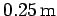. At a point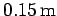away from the equilibrium position, the velocity of the block is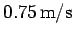. What is the period of oscillation of the block?

Answer: The equation of simple harmonic motion iswhereis the displacement, andis the amplitude. We are told that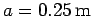. The velocity of the block is obtained by taking the time derivative of the above expression: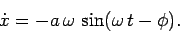We are told that at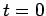(say),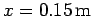and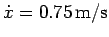. Hence,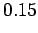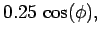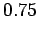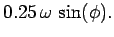The first equation gives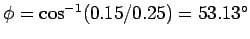. The second equation yields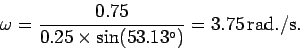Hence, the period of the motion is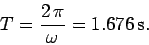Next: Worked example 11.3: Block Up: Oscillatory motion Previous: Worked example 11.1: Piston
Richard Fitzpatrick 2006-02-02